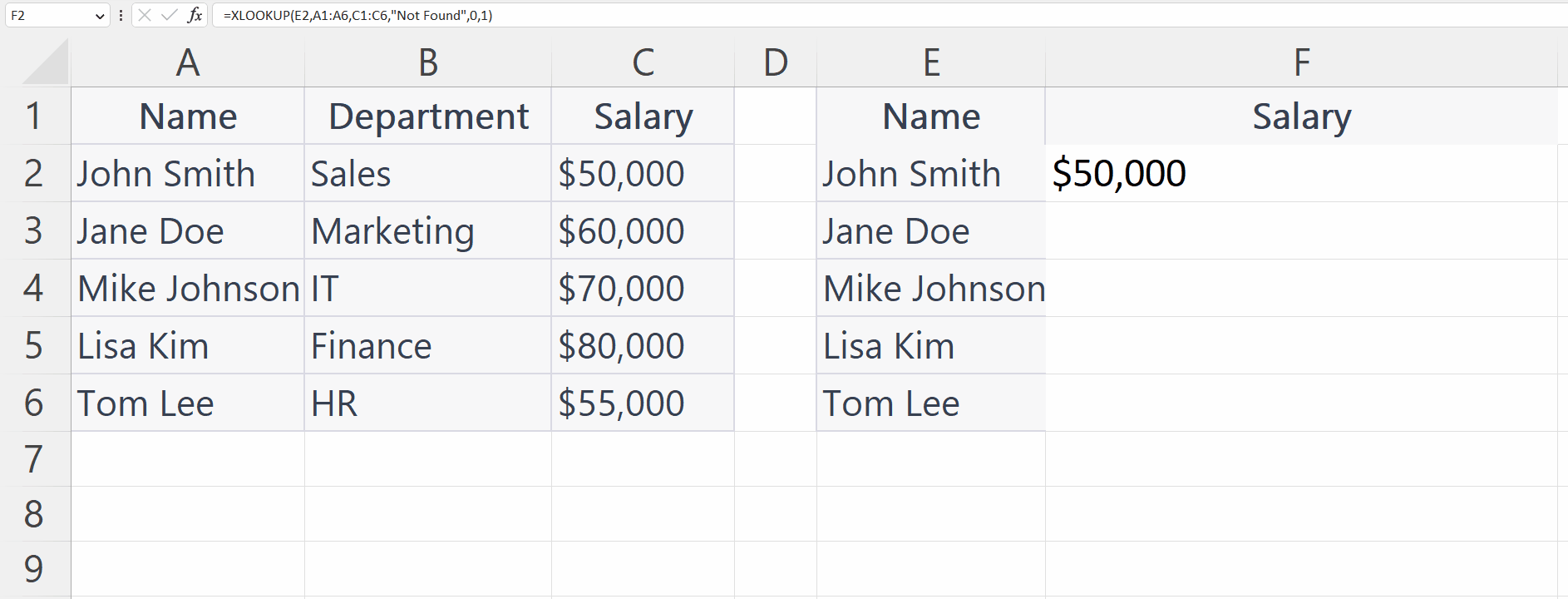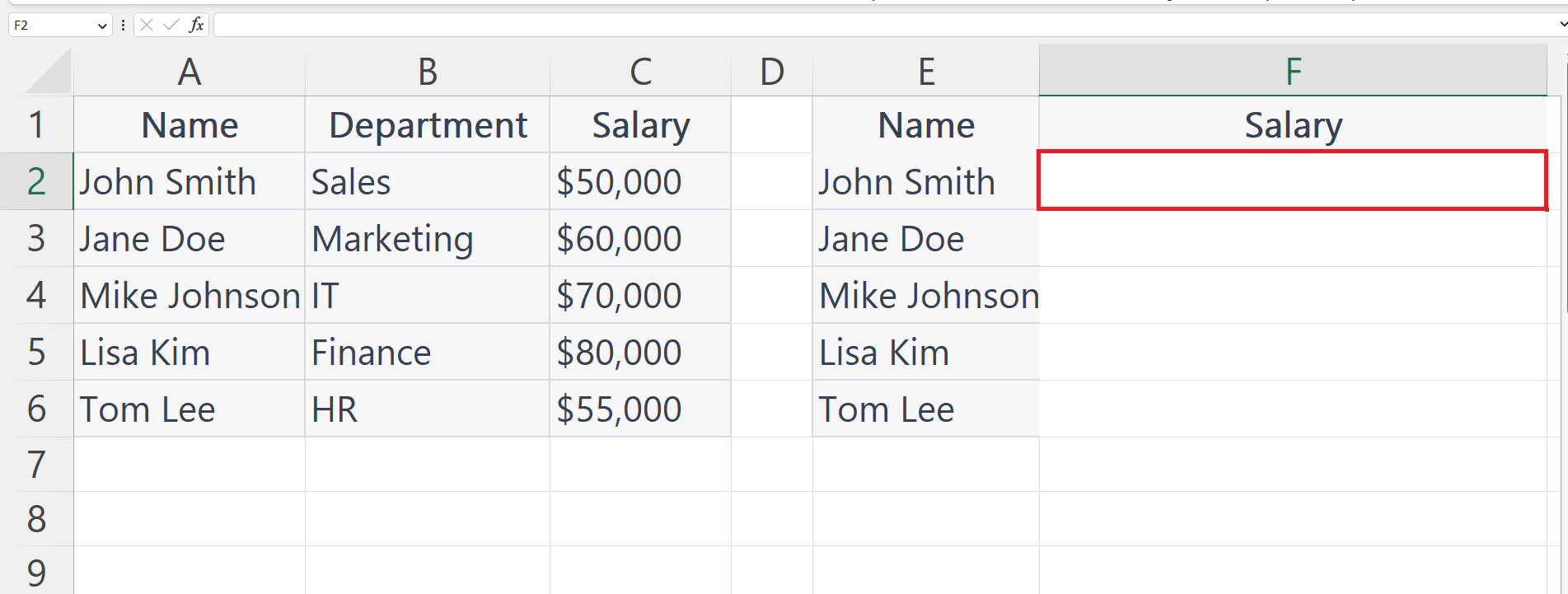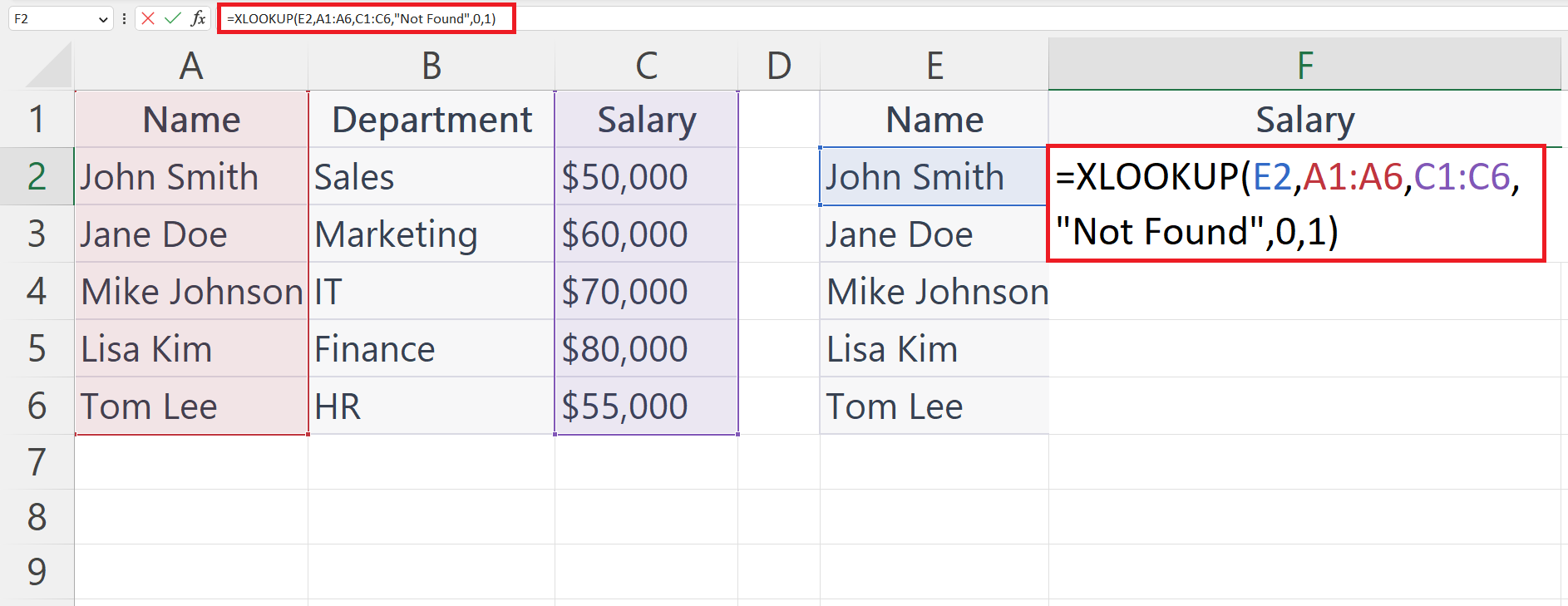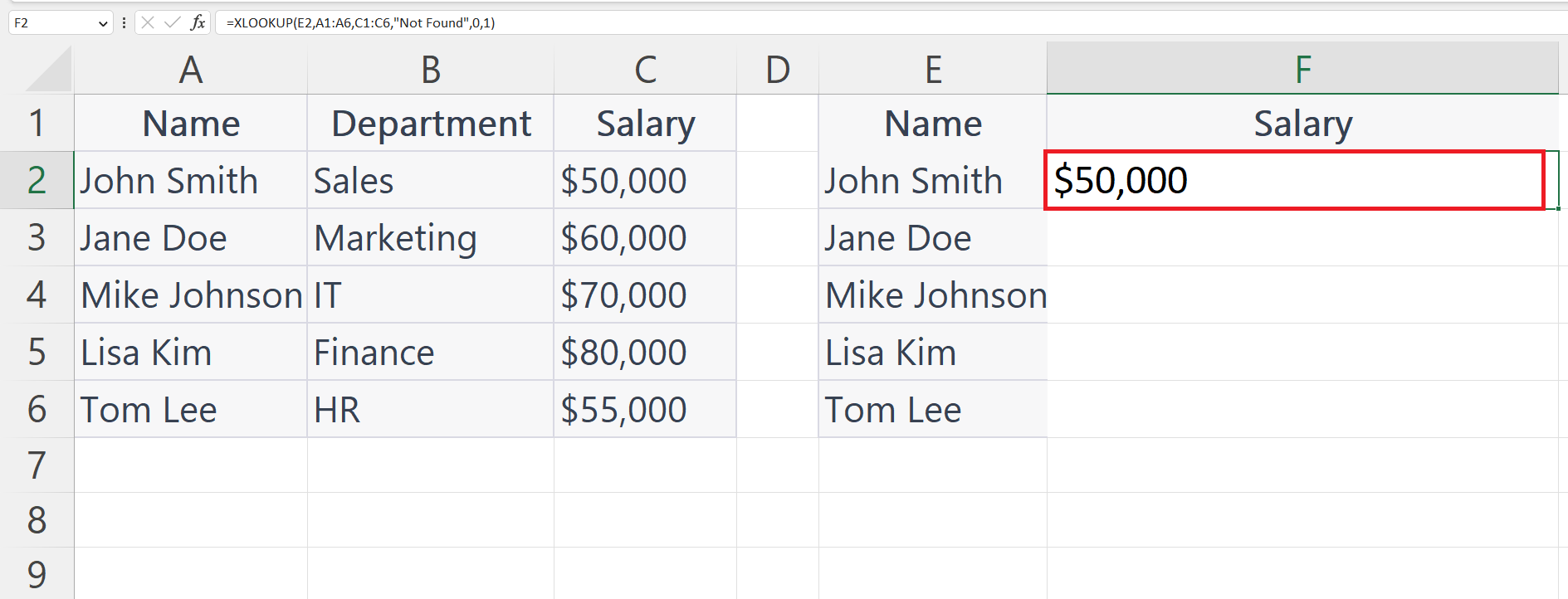# How does XLOOKUP function work in Microsoft Excel

In this tutorial we will learn how XLOOKUP function works in Microsoft Excel. The XLOOKUP function in Excel is used to find a particular value in a table or array and then return a corresponding value from another column in the same table or array. Let’s understand the syntax of XLOOKUP function first.

## Syntax of XLOOKUP

The basic syntax of the XLOOKUP function is as follows:

=XLOOKUP(lookup_value, lookup_array, return_array, [if_not_found], [match_mode], [search_mode])

The XLOOKUP function requires at least three arguments: lookup_value, lookup_array, and return_array. The match_mode and search_mode arguments are optional.

Lookup Value: The first argument, lookup_value, is the value that you want to search for. This value can be a number, text, or a cell reference.

Lookup Array: The second argument, lookup_array, is the range or array of cells that you want to search in. The lookup_array must have at least two columns: one column for the lookup values, and another column for the return values.

Return Array: The third argument, return_array, is the range or array of cells that contains the values that you want to return. The return_array can have one or more columns.

[if_not_found]: You can add an optional argument to the XLOOKUP function to specify what should happen if the lookup_value is not found in the lookup_array. This argument is called [if_not_found] and can be added as the last argument in the function.

Match Mode: The fourth argument, match_mode, is optional and specifies the type of match you want to use. There are four options:

• 0 or omitted: Exact match (default)
• 1: Exact or next smallest match
• -1: Exact or next largest match
• 2: Wildcard match

Search Mode: The fifth argument, search_mode, is optional and specifies whether to search for the lookup_value from the beginning or end of the lookup_array. There are two options:

1 or omitted: Search from the beginning (default)

-1: Search from the end

The XLOOKUP function returns the value that corresponds to the lookup_value in the return_array. If the lookup_value is not found in the lookup_array, the function can return an optional default value or an error message.

Here we have a dataset above showing Department and Salary of some employees. We will extract the salary of one of the employees i.e.John Smith from the data using the XLOOKUP function.XLOOKUP is a powerful lookup function in Excel that allows you to search for a value in a range or an array and return a corresponding value from the same position in a different range or array. The XLOOKUP function is a versatile tool for data analysis and can save you time and effort when working with large datasets in Excel.

### Step 1 – Select a Blank Cell– Select a blank cell in which you want to extract the data.

### Step 2 – Place an Equals Sign### Step 3 – Use the XLOOKUP Function– Use the XLOOKUP function to extract the data from the data range.

### Step 4 – Press the Enter Key– Press the Enter key to extract the data.

### Step 5 – Use Autofill to Extract Data for Each Employee– Use the Autofill feature to extract data for each employee.